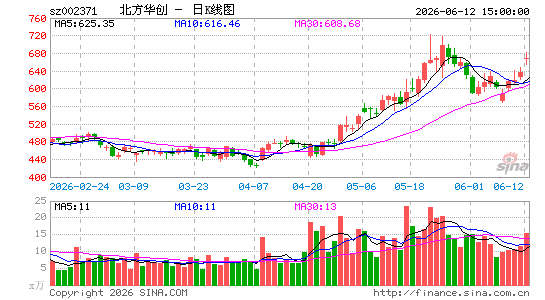•北方华创

-----------------------------------------------
VAR1:=EMA(HHV(HIGH,500),21);
VAR2:=EMA(HHV(HIGH,250),21);
VAR3:=EMA(HHV(HIGH,90),21);
VAR4:=EMA(LLV(LOW,500),21);
VAR5:=EMA(LLV(LOW,250),21);
VAR6:=EMA(LLV(LOW,90),21);
VAR7:=EMA((VAR4*0.96+VAR5*0.96+VAR6*0.96+VAR1*0.558+VAR2*0.558+VAR3*0.558)/6,21);
VAR8:=EMA((VAR4*1.25+VAR5*1.23+VAR6*1.2+VAR1*0.55+VAR2*0.55+VAR3*0.65)/6,21);
VAR9:=EMA((VAR4*1.3+VAR5*1.3+VAR6*1.3+VAR1*0.68+VAR2*0.68+VAR3*0.68)/6,21);
VARA:=EMA((VAR7*3+VAR8*2+VAR9)/6*1.738,21);
VARB:=REF(LOW,1);
VARC:=SMA(ABS(LOW-VARB),3,1)/SMA(MAX(LOW-VARB,0),3,1)*100;
VARD:=EMA(IF(CLOSE*1.35<=VARA,VARC*10,VARC/10),3);
VARE:=LLV(LOW,30);
VARF:=HHV(VARD,30);
VAR10:=IF(MA(CLOSE,58),1,0);

---------------------------------------------------------